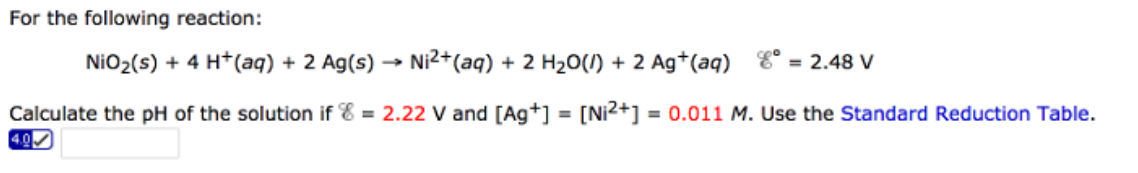# Problem: For the following reaction:NiO2(s) + 4 H+ (aq) + 2 Ag(s) → Ni2+      E°= 2.48 VCalculate the pH of the solution if E = 2.22 V and [Ag+] = [Ni2+] = 0.011 M. Use the Standard Reduction Table.

###### FREE Expert Solution
95% (88 ratings)###### Problem Details

For the following reaction:

NiO2(s) + 4 H+ (aq) + 2 Ag(s) → Ni2+      E°= 2.48 V

Calculate the pH of the solution if E = 2.22 V and [Ag+] = [Ni2+] = 0.011 M. Use the Standard Reduction Table.